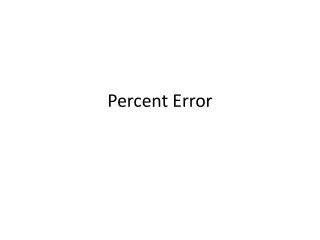DownloadDownload PresentationPercent Error

# Percent Error

Télécharger la présentation## Percent Error

- - - - - - - - - - - - - - - - - - - - - - - - - - - E N D - - - - - - - - - - - - - - - - - - - - - - - - - - -
##### Presentation Transcript

1. Percent Error

2. Percent Error The percent errorcan be determined when the experimental value is compared to the accepted valueaccording to the equation below:

3. What you got.

4. A percent error can be either positive or negative. If the measured value is greater than the accepted value, the difference (measured value – accepted value) will be positive, and the percent error will be positive. If the measured value is less than the accepted value, the difference will be negative and the percent error will be negative.

5. Example: The accepted value for the density of copper is 8.93 g/cm3. In a laboratory setting, three students are asked to experimentally determine the density of copper. Their results are Student 1: 8.91 g/cm3 Student 2: 8.23 g/cm3 Student 3: 8.99 g/cm3 Calculate the percent error associated with each student’s reported density.

6. Experimental value: Student 1: 8.91 g/cm3 Accepted value: 8.93 g/cm3 - ( ) 8.91 g/cm3 8.93 g/cm3 X 100 = -0.2% error 8.93 g/cm3 Always put a box around your answer. Note that there are no units associated with percent error; g/cm3, present in both numerator and denominator, cancel.

7. Experimental value: Student 2: 8.23 g/cm3 Accepted value: 8.93 g/cm3 - ( ) 8.23 g/cm3 8.93 g/cm3 X 100 = -7.8%error 8.93 g/cm3 Always put a box around your answer.

8. Experimental value: Student 3: 8.99 g/cm3 Accepted value: 8.93 g/cm3 - ( ) 8.99 g/cm3 8.93 g/cm3 X 100 = 0.7% error 8.93 g/cm3 Always put a box around your answer.

9. Problems • 1. A student calculated the volume of a test tube to be 10.3 mL. The true volume is 10.0mL. - ( ) 10.3 mL 10.0 mL X 100 = 3% error 10.0 mL

10. 2. A student determines the density of gold to be 18.98 g/L. The true density of gold is 19.30 g/L. - ( ) 18.98 g/L 19.30 g/L X 100 = -1.66% error 19.30 g/L

11. 3. An equation was solved using 299,000,000 m/s as the speed of light. Actually the speed of light in a vacuum is exactly 299,792,458 m/s. - ( ) 299,000,000 m/s 299,792,458 m/s = X 100 -0.26434 % error 299,792,458 m/s

12. 4. A group of students using a triple beam balance found the mass of a copper sample to be 2.40 g. The actual mass of the copper sample is 2.41 g. - ) ( 2.41 g 2.40 g X 100 = -0.415% error 2.41 g

13. 5. Working in the laboratory, a student find the density of a piece of pure aluminum to be 2.85 g.  The accepted value for the • density of aluminum is 2.69 g. - 2.69 g ) ( 2.85 g X 100 = 5.95% error 2.69 g

14. 6. A group of students using a triple beam balance found the mass of an aluminum sample to be 1.90 g. The actual mass of the aluminum sample is 2.01 g. - 2.01 g ) ( 1.90 g X 100 = -5.47% error 2.01 g

15. 7. A student experimentally determines the specific heat of water to be 4.29 J/g Co.  He then looks up the specific heat of water on a reference table and finds that is 4.18 J/g Co.    - 4.18 J/g ) ( 4.29 J/g X 100 = 2.63% error 4.18 J/g

16. 8. A student takes an object with an accepted mass of 200.00 grams and masses it on his own balance.  He records the mass of the object as 196.5 g. - 200.00 g ( ) 196.5 g X 100 = -1.75% error 200.00 g

17. 9. A student measured the length of a line to be 23 cm long. The actual length of the line is 23.101 cm long. - 23.101 cm ( ) 23 cm X 100 = -0.437% error 23.101 cm

18. 10. A person was traveling 65 mph in a 55 mph zone. After being stopped by an officer, the person claimed his speedometer said 55 mph not 65 mph. - 55 mph ( ) 65 mph X 100 = 18% error 55 mph